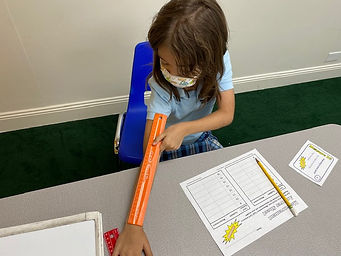## Ms. Dawn

### Target 1​

###### Lesson Type:

New

Measurment

:

Comparison

Measure and compare objects in standard units.

###### 1:

Understand how to measure length using a standard unit (centimeter, inches).

###### 2:

Measure the length of objects by selecting appropriate measurement tools.

###### 3:

Estimate length based on understandings of unit measurements.

###### 4:

Identify that length can be measure with rulers, tape measures, etc.

###### 5:

Understand equivalent measurements.

3rd

###### Vocabulary:

Length, Centimeter, Inches, Convert, Foot, Equivalent, Measurement tools, Estimate,

Activities:

1. Students read a variety of task cards and decided what measurement tool they will use and what unit of measurement is the most appropriate. Then, they estimated each item and finally found the exact measurement.

2. Students used strategies and their understanding to convert measurements (Inches to feet and feet to inches).### Home Exploration

###### Guiding Questions:## Absent Students:

### Target 2

:

###### 1:

Identify the unknown information in problems.

3rd

###### Vocabulary:

Variable, Unknown, Represent, Equation

Activities:

1. Students were given a variety of equations with different operations and found the unknown number.

2. Students worked their way up from bronze, silver, and gold problems. They used a variety of strategies and showed or verbally communicated their thinking.### Home Exploration

###### Guiding Questions:### Target 3

:

###### Vocabulary:

Activities:### Home Exploration